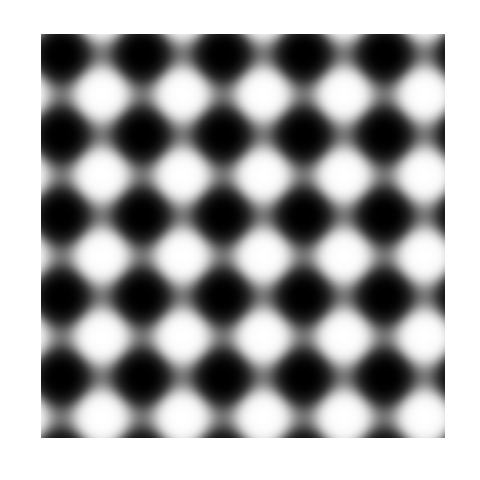# Trigonometric Pattern Design

Triangles are my favorite shape, three points where two lines meet (Tessellate, Alt-J)

Inspired by recurrence plots and by the Gauss error function, I have done the following plots. The first one represents the recurrence plot of$f\left ( x \right )= sec\left ( x \right )$ where distance between points is measured by Gauss error function:This one is the same for$f\left ( x \right )= tag\left ( x \right )$And this one represents$latex f\left ( x \right )= sin\left ( x \right )$I like them: they are elegant, attractive and easy to make. Try your own functions. One final though: the more I use magrittr package, the more I like it. This is the code for the first plot.

library("magrittr")
library("ggplot2")
library("pracma")
RecurrencePlot = function(from, to, col1, col2) {
opt = theme(legend.position  = "none",
panel.background = element_blank(),
axis.ticks       = element_blank(),
panel.grid       = element_blank(),
axis.title       = element_blank(),
axis.text        = element_blank())
seq(from, to, by = .1) %>% expand.grid(x=., y=.) %>%
ggplot( ., aes(x=x, y=y, fill=erf(sec(x)-sec(y)))) + geom_tile() +